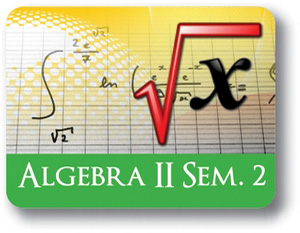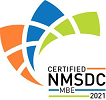Red Comet## Algebra II - Semester - 2

#### Course Price: \$285.00## Course Details:

This course delves into the concepts of Exponentials, e, Logarithm and anti-logarithm. The course develops on the properties of logarithm to solve exponential and logarithm equations and graph logarithm and exponential functions. The course moves on to the concepts of Trigonometry. The course deals with the properties of Sine, Cosine and Tan functions, relationship between the trigonometric functions, Pythagorean theorem. This part of the course deals with finding the sine, cosine and tan value of in a right-angle triangle. The course moves on to the concepts of the arithmetic and geometric series. Finding the nth term and sum of all terms in each of the series are discussed in this section. Using these concepts problems are solved for finding unknown variables in a given series. The course moves on to the concepts involving conic sections – circles and parabolas. The course deals with the concepts of graphing – how to identify and graph a given function, behavior of various functions though graphing, relationship between functions through graphing, and solving functions through graphing.

Note: This course is not designed for ELL (English Language Learners) students. ELL students may enroll in this course ONLY if they have adequate mentor support at their home school and are able to fulfill all course requirements.

## Syllabus:

Section 1: Logarithm and Exponential Functions

• Recap: Exponent and Exponent Rules
• Exponential Growth and Decay
• Introduction to e and Logarithms
• Logarithm, Antilogarithm Tables and e
• More Logarithmic Properties
• Solving Exponential Functions with Logarithm
• Graphs of Exponential and Logarithmic Functions

Section 2: Trigonometry

• What is Trigonometry?
• Graphs Of Basic Trigonometric Functions
• Basic Trigonometric Identities
• Sinusoidal Graphs

Section 3: Graphing and Interpreting Data

• Introduction to Representing Data
• Solving Systems by Graphing
• Interpreting and Comparing Graph Features
• Graph Functions
• Causation and Correlation
• Graphing Linear Inequalities
• Graphs of Exponents and Logarithms
• Modeling with Functions
• Interpreting Data: Statistics

Section 4: Sequences and Series

• Introduction to Sequences, Series and Series Notation
• Arithmetic Series
• Applications of Arithmetic Series
• Geometric Series
• Applications of Geometric Series
• Recursive Sequences

Section 5: Conic Sections

• Introduction to Conic Sections
• Features and Standard Equation of Circle
• Parabolas

### Accreditation & Approvals